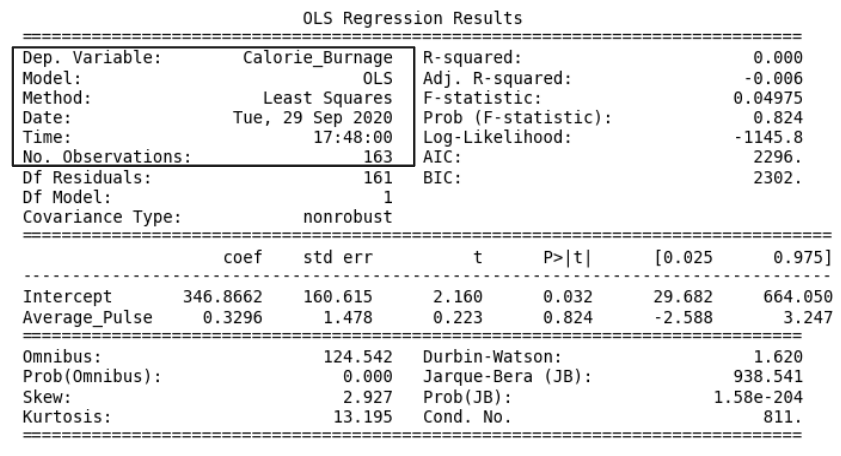# Data Science - Regression Table - Info

## The "Information Part" in Regression Table• Dep. Variable: is short for "Dependent Variable". Calorie_Burnage is here the dependent variable. The Dependent variable is here assumed to be explained by Average_Pulse.
• Model: OLS is short for Ordinary Least Squares. This is a type of model that uses the Least Square method.
• Date: and Time: shows the date and time the output was calculated in Python.